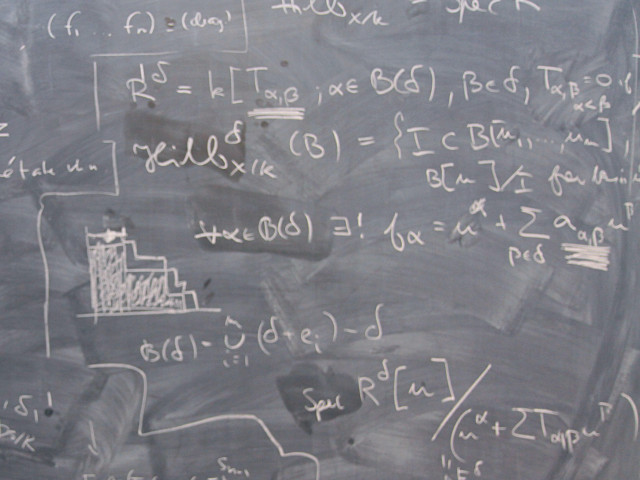Course offering missing for current semester as well as for previous and coming semesters

## Content and learning outcomes

### Course contents *

• Basic algebra.
• Equations and inequalities.
• Functions.
• Rational functions, partial fractions.
• Logarithmic and exponential functions.
• Trigonometric functions.
• Introduction to vectors.
• Scalar and vector product.
• The line and the plane.
• Matrices and determinants.
• Linear equations, gauss elimination.
• Limits.
• Differentiation, common derivatives, rules of differentiation.
• Extremal values, asymptotes, graph sketching.
• The antiderivative, definite and indefinite integrals.
• Methods of integration.
• Improper integrals.
• Applications of integrals.

### Intended learning outcomes *

The course is intended to consolidate and deepen the students knowledge in algebra and single variable calculus, and to introduce vector geometry and linear algebra.

To obtain the grade E, the student should be able to:

• simplify and factorise algebraic expressions and to calculate partial fractions.
• solve equations and inequalities and to and handle expressions including absolute values.
• carry out and interprete vector geometrical operations including angles, distances, projections, lines and planes in 3D.
• solve sets of linear equations using gauss elimination.
• carry out matrix operations and to solve simple matrix equations.
• calculate 2x2- and 3x3-determinants och inverses to 2x2-matrices and to use Cramers rule to solve simple linear systems.
• state the existence of solutions of linear systems by examining the coefficient matrix.
• to use, describe and interprete the most common functions, and their inverses, in the engineering sciences,.
• calculate simple limits.
• define the derivative of a function, to give examples of technical applications, and to use derivates of common functions.
• use the chain, product and quotient rules, as well as logarithmic and implicit differentiation.
• determine the properties of functions by calculating their asymptotes and extremal values.
• use common antiderivatives to calculate definite and indefinite integrals.
• apply integration by parts, integration by substitution, and integration of rational functions.
• calculate simple improper integrals.
• interprete and use integrals in calculating areas in graphs and volumes of solids of revolution.

To obtain higher grades, the student should also be able to:

• calculate determinants and inverses of 3x3 matrices and larger.
• theoretically prove some of the theorems and rules used in the course.
• deduce the derivatives used in the course.
• solve more demanding mathematical problems in the course areas.
• interprete and use integrals in further applications, e g in calculating arclengths, centres of gravity and moments of inertia.
• analyse and structure more complicated problems, to use appropriate mathematical models and solution methods, and to interprete results.

### Course Disposition

No information inserted

## Literature and preparations

### Specific prerequisites *

Knowledge corresponding to admission requirements for Bachelor of Science in Engineering.

Basic experience of computers.

### Recommended prerequisites

No information inserted

### Equipment

No information inserted

### Literature

The course literature is posted on the course’s homepage at least four weeks before the course starts.
The previous academic year used the following literature:
Rodhe & Sollervall, Matematik för ingenjörer. (5:e uppl) KUB 2001.

## Examination and completion

If the course is discontinued, students may request to be examined during the following two academic years.

A, B, C, D, E, FX, F

### Examination *

• TEN1 - Examination, 7.5 credits, Grading scale: A, B, C, D, E, FX, F

Based on recommendation from KTH’s coordinator for disabilities, the examiner will decide how to adapt an examination for students with documented disability.

The examiner may apply another examination format when re-examining individual students.

### Other requirements for final grade *

Passed written exam

### Opportunity to complete the requirements via supplementary examination

No information inserted

### Opportunity to raise an approved grade via renewed examination

No information inserted

### Ethical approach *

• All members of a group are responsible for the group's work.
• In any assessment, every student shall honestly disclose any help received and sources used.
• In an oral assessment, every student shall be able to present and answer questions about the entire assignment and solution.

## Further information

### Course web

Further information about the course can be found on the Course web at the link below. Information on the Course web will later be moved to this site.

Course web HN1901

### Offered by

ITM/Sustainable Production Development

### Main field of study *

Mathematics, Technology

First cycle